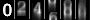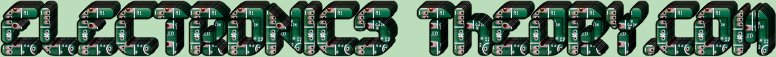# Lesson 51 - Filter Circuits

So far, we have discussed the basic electronic components, and power supply circuits. We have touched on filters, but not discussed them in detail. No course on electronics would be complete without a thorough understanding of filters, how they work, and how to recognize them when you see them.

Filters are used in almost every type of electronic device, but are possibly the least understood part of the device. We all have a basic understanding of what a filter is, but to study them in detail - now THAT is a study indeed! This course does not intend to make you an expert in designing microwave frequency filters, but does intend to give you enough overview to recognize one, and to repair one if you need to.

To begin with, lets go back to our old friend - water. We all understand what a water filter is. It lets the stuff we want ( the water ) pass through it, but blocks the other flotsam and jetsam that we don't want to drink, swim in, etc. Air filters and oil filters work in a similar manner. They pass the good stuff, and block out the bad stuff. Well - filters in electronics work in the same basic manner, albeit an understanding of the discrete components will go a long way to understanding how they work.

You've already touched on the basic filter devices - the capacitor and the coil. You already have a simplistic understanding of how these devices work. Now lets look at each in more detail.

The Capacitor:

The capacitor, in simplistic terms, passes AC and blocks DC. In short - this is the definition of a filter, no? In reality - a capacitor is not quite so simplistic. Recall we talked about capacitors having a "TIME CONSTANT". If you change the capacitance, you change the time constant. Well, there is a specific relationship between time and frequency, where Frequency is the reciprocal of time ( T=1/F and F=1/T ). So if you change the value of the capacitor, you also change the FREQUENCY of the circuit the capacitor is in!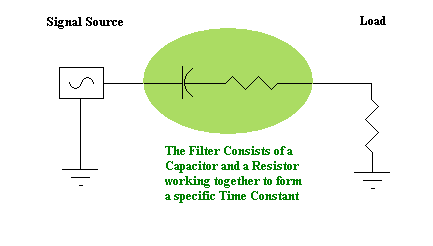To put that in other words - "Capacitors pass AC" is sort of a blanket statement, albiet not completely true. A given capacitor will pass all frequencies ABOVE A CERTAIN FREQUENCY, and block all signals BELOW that frequency. So the capacitance value of a given circuit is chosen to allow a certain range of frequencies to pass through. Let's give a few examples: The time constant formula for capacitors is "T=RC" or Time = Resistance x Capacitance. If we take a circuit with a resistance of 10 Ohms, and a capacitance of 10uF, then the Time would be 0.0001 seconds. The Frequency would be 1/.0001, or 10,000 Cycles Per Second ( also called Hertz ). So a 10 Ohm resistor and a 10uF capacitor would yield a circuit passing 10 KHz.

Let's try another one.

This time, we have a 100 Ohm resistor, and a 25 picoFarad (pF) capacitor. I understand that you may have forgotten what all the nano's pico's, and micro's stand for, so I've included this little table for a help:
 Prefix Analogvalue p (pico) 10-12 n (nano) 10-9 µ (micro) 10-6 m (milli) 10-3 k (kilo) 103(1000) M (mega) 106(1,000,000) G (Giga) 109(1,000,000,000)
So a 25 pF capacitor would be 25x10-12 power or 0.000000000025 Farads. Now we multiply that times 100 Ohms, and come up with a time constant of 0.0000000025 seconds. We now take the reciprocal ( 1 / 0.0000000025 ) comes to 400000000 Cycles per Second (CPS/Hertz) or 400 MegaHertz (MHz).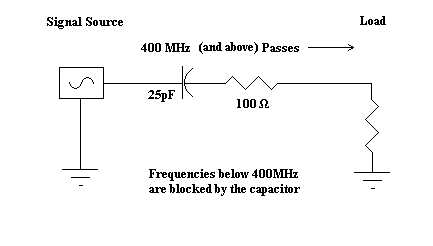Now comes the tricky part - where does it pass those signals to? That depends entirely on the capacitor's placement in the circuit. If the capacitor is placed in SERIES with the signal flow - it will allow the 400 MHz signal to PASS to the next stage.

If, however it is placed in Parallel to the signal flow - it will pass the 400 MHz signal to ground - effectively BLOCKING the signal from passing to the next stage.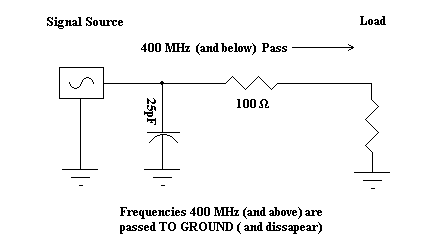So the placement of the capacitor is KEY in whether the signal is passed, or blocked. Note that when the higher frequencies are passed to ground ( essentially blocked from the normal signal path ), the lower frequencies are allowed to go free, and hence are passed to the rest of the circuit.

I smell a memory aid here! If the capacitor is "High" on the schematic ( in series with the signal flow ), it is a High Pass Filter (passes high frequencies). If however, the capacitor is "Low" on the schematic ( in parallel with the signal flow ), it is a Low Pass Filter (passes low frequencies). High Cap = High Pass, Low Cap, Low Pass.

Note also that the "cut off" frequency can be changed in either of two ways - by changing the value of the capacitor, or by changing the value of the resistor.

All that being said and done - let's examine a different scenereo. We said earlier that filters can be made using capacitors OR COILS. What happens if we replace the capacitor with the coil? Well, the first thing we encounter is a slightly different formula. Remember that coils operate in almost a completely opposite manner than does a capacitor. It's like they are yin and yang or something. The formula for the capacitor's time constant is T=RC. The formula for the Coil's time constant is "T=R/L", and without going into all the math ( we hate math don't we? ), we can simply assume ( and correctly so ) that the coil reacts almost opposite to the way the capacitor does. What exactly do I mean by that?

When we put a coil in SERIES with the signal flow ( recall signal flow goes from left to right ), it passes low frequencies and blocks higher frequencies. When we put a coil in Parallel with the signal flow, it passes those same low frequencies to ground, and blocks the higher frequencies from ground ( in effect passing those high frequencies through the signal path, and sending the lows to the abyss. )

Do how does this work in the real world? Let's look at our Power Supply circuit as an example.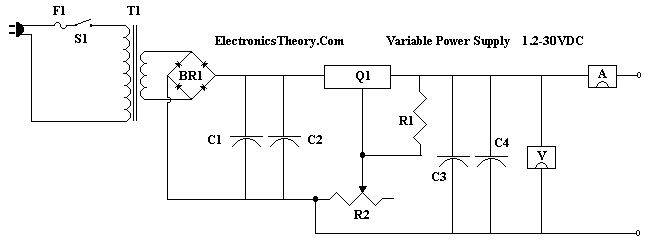In this circuit, we have 2 capacitors following the rectifier. Those two capacitors are there to PASS the HIGH frequencies ( in this case ANY AC ) to ground - effectively eliminating it, while allowing the lower frequencies (DC) to pass on to the regulator unaffected. We could improve on this design by making the following change: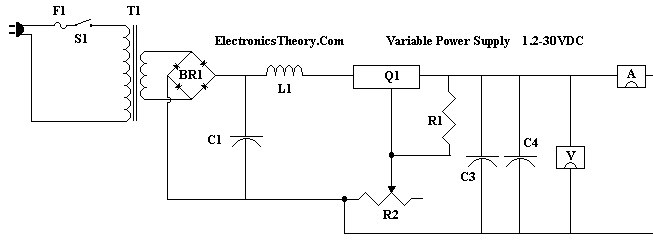Note that we have removed C2, and replaced it with L1. The capacitor C1 will still take the higher frequencies, and pass them to ground, but now we have an added advantage - L1 will BLOCK any high frequencies that may have tried to get by C1. At the same time, C1 will keep our low frequencies from disappearing into ground, while L1 will happily pass our lower frequencies and let them go right into the regulator. The only question, then, is what values to use with our Capacitor and Inductor, to pass the right stuff, and block the bad stuff. First we have to decide what is the bad stuff. The bad stuff, that we don't want getting through, is the line frequence of the power lines. In the United States - that would be 60 Hertz. In other countries, it might be 50 Hertz. Either way, if we make the "CUT-OFF" frequency of our filter 20 Hertz - the line ripple will be forced into the dirt, and the DC component of our signal will pass through unaffected. You might ask why 20 Hertz and not 40? Well, you have 2 peaks within 60 Hertz. The first is at 30, and the second is at 60. We want to eliminate BOTH of them. ( This is a high dollar, well designed power supply ). Being given the cut-off frequency of 20 Hz, we have a good starting point.

Recall that any time you use a coil and a capacitor together, you have both Inductive Reactance (resistance of the coil to AC), and Capacitive Reactance (resistance of the Capacitor to AC) to contend with. We also have a magic combination called "Resonance", wherein the Capacitive Reactance is EQUAL to the Inductive Reactance (XC = XL). We know from the previous page that the capacitor C1 is equal to 14000uF. We also know the formula for Capacitive Reactance ( What? You haven't memorized it by NOW??) is XC=1/(2πfC). So we can solve for XC!

XC=1 / 2 * π * frequency * C
XC=1 / 2 * π * 60 * C
XC=1 / 6.28 * 60 * C
XC=1 / 6.28 * 60 * C
XC=1 / 376.8 * C
XC=1 / 376.8 * 14000uF
XC=1 / 376.8 * .014F
XC=1 / 5.2752
XC=0.19 Ohms
So at 60 Hz, this capacitor has less than .2 Ohms of resistance.... it's near a dead short! But what if this same capacitor were given, say 2 Hertz signal ( near DC )?
XC=1 / 2 * π * frequency * C
XC=1 / 2 * π * 2 * C
XC=1 / 6.28 * 2 * C
XC=1 / 6.28 * 2 * C
XC=1 / 12.56 * C
XC=1 / 12.56 * 14000uF
XC=1 / 12.56 * .014F
XC=1 / .176
XC=5.6 Ohms
Boy do I hate math.... but sometimes it is necessary to prove a point - and that point being - that as the frequency decreases - the resistance increases. If then, the frequency were reduced to, say ZERO (Pure DC), then the resistance, in theory, would be increased to INFINITY. Of course, we'll never know, because you can't divide by ZERO, but you can divide by something close to zero, say .0000000000005, which yields a very big resistance!

Now that we have the Capacitive Reactance of the capacitor at 60 Hertz, we need to find a coil with an equal inductive reactance at that same frequency!. The math is tedious, but not impossible.
XL=XC
XL=0.19 Ohms
XL=2 * π * frequency * L
XL=6.28 * frequency * L
XL=6.28 * 60 * L
XL=376.8 * L
and since we already know that XL=0.19 Ohms, we can say:

.19 Ohms = 376 * L !!

So if we divide both sides of the equal sign by 376, we get -

L = 5 x 10-4 or
L = .0005 Henries ( 5mH )

EXCELLENT!!!

Except for 1 thing -

We solved the problem for 60 Hertz, and we wanted to solve it for 20 Hertz!
oops.
It's HOMEWORK time!
I won't MAKE you do the math - but if you really want to understand this, math is important to electronics - so you'll have to solve it for yourself and find out WHY we use a coil with HIGHER inductance than 5mH for this circuit ( and find out how many Henries we would use ).

 (On The Following Indicator... PURPLE will indicate your current location) 1 2 3 4 5 6 7 8 9 10 11 12 13 14 15 16 17 18 19 20 21 22 23 24 25 26 27 28 29 30 31 32 33 34 35 36 37 38 39 40 41 42 43 44 45 46 47 48 49 50 51 52 53 54 55 56 57 58 59 60 61 62 63 64 65 66 67 68 69 70 71 72 73 74 75

[COURSE INDEX] [ELECTRONICS GLOSSARY] [HOME]Otherwise - please click to visit an advertiser so they know you saw their ad!

VISITORS: